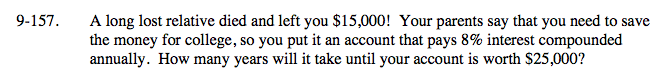### Home > A2C > Chapter 9 > Lesson 9.3.2 > Problem9-157

9-157.Remember the annual compound interest formula.
A = P(1 + r)t

First substitute the values into the equation.
25000 = 15000(1.08)t

Divide both sides by 15000.

$\frac{5}{3}=(1.08)^{\textit{t}}$

Remember the rules of logarithms and take the log of both sides.

$\text{log}\left(\frac{5}{3}\right)=\textit{t}(\text{log}1.08)$

Solve for t.

The account will be worth 25000 in between 6 and 7 years.

t = 6.64